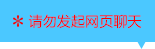| | |

||
APP端下载AndroidiPhone
￥198

|
2033人点赞
586538人已学习
|

3天无理?#36175;?#27454;

3天无理?#36175;?#27454;?#21644;?#27454;将以超级币形式退至您的超级课堂学习账户，便于您重新选购其他课程。恶意退款将被冻结账号。

• 1、复合函数的定义，要弄清楚外层函数，内层函数，直接变量，中间变量，因变量这些概念
2、 我们看待复合函数的方式，只是为了更好的研究它，其中中间变量是拆卸和组装复合函数的关键
3、 注意“内层函数的函数值，是外层函数的自变量?#20445;?#25152;?#38405;?#23618;函数的值域与外层函数的定义域的交集必须非空
4、 在做题时，可以用“换元法”的视角来求复合函数的解析式，将内层函数整体代入
• 1、两种基本的图像变换—关于$y$轴的对称变换和$x$轴方向上的平移变换。前者很简单，只要把$x$变成$-x$就$OK$，后者要注意“孤立$x{}$"，看它的变化，遵循左加右减的规律。?#27604;?#20320;?#37096;?#20197;使用公式
2、 ?#39068;?#20004;?#30452;?#25442;综合起来，可?#22278;?#29992;两步走策略。“先对称，再平移?#34987;頡?#20808;平移，再对称?#20445;?#20004;种方式异曲同工
3、 图像变换，我们之后还会遇到更多与之相关的知识点。可?#36816;?#20989;数的所有性质，都可以结合图像进行分析，图像就相当于函数的人格另一面，更深刻形象地反应出其中蕴含的数学意义
• 1、“括号同级原理”$f(a)$中的$a$和$f[g(a)]$中的$a$根本不是同一个$a$，根据“括号同级原理?#20445;?#21069;者$a$相当于后者的$g(a)$$f[g(a)]$的求法，如果已知复合函数的解析式，就直接带入。如果给出外层和内层函数$f(x)$与$g(x)$各自的解析式。你就有两种选择：(1)、把复合函数组装起来，再代入。(2)、先代入内层函数求值，再代入外层函数求值。反过来，我们也能通过$f[g(a)]$的值，求$a$值，或者求未知参数
2、 $f(a)$的两种基本求法：(1)解$x$代入法?#21644;?#36807;$g(x)=a$解出$x$的值，再代入复合函数的解析式；(2)整体代入法?#21644;?#36807;代数变形，把复合函数用内层函数的整体表示，直接带入求值
• 1、“括号?#27573;?#30456;同”这个重要的结论，围绕它主要有三种题型：已知$f(x)$的定义域，求$f[g(x)]$的定义域。已知$f[g(x)]$的定义域，求$f(x)$的定义域。已知$f[g(x)]$的定义域，求$f[h(x)]$的定义域。
2、 抓住“括号?#27573;?#30456;同”这个法则，就能在各种函数的定义域之间切换自由
• 1、已知解析式，由内向外，逐层求值域。对于二次函数和反比例函数，最?#38376;?#21512;图像分析值域。如果人为规定了定义域，也是?#20945;?#20010;顺序算。反过来，规定了值域，也能?#36175;?#21521;内求解集
2、 若解析式未知，对于$f[g(x)]$与$f(x)$，若括号?#27573;?#30456;同，即$g(x)$的值域和$x$的定义域相同，则$f[g(x)]$与$f(x)$的值域相同。若前者不同，后者的值域是不确定的，可能相同，?#37096;?#33021;不同
• 1、形如$f(x)=\frac{p(x)}{q(x)}$叫做分式函数，其中$p(x)$、$q(x)$是既约整式且$q(x)$的次数不低于一次。其中形如$f(x)=\frac{(ax+b)}{(cx+d)}$的是一次分式函数。注意$c\neq 0$，且$ad\neq bc$
2、 分子为常数的一次分式函数，可由反比例函数的图像横向平?#39057;?#21040;；分子有自变量的一次分式函数，要用分离常数法把解析式恒等变形为常数分离的形式，再考虑横向和纵向的平移
3、 分离常数法的经典步骤。第(1)步：分子分母系数化$1$。第(2)步：在分子中制造约分项。注意恒等变形。第(3)步：约分，分离常数
4、 函数平移后对称中心的确定，由于?#30333;?#21152;右减?#20445;?#23545;称中心的横坐标要加负号。当解析式不是常数分离的形式时，要用分离常数法处理，才能?#19994;?#23545;称中心。
• 1、对于一般的一次分式函数，用分离常数法处理后，求出其对称中心，在反比例函数定义域和值域的基础上，用横坐标代替$0$，写出定义域；以纵坐标代替$0$，写出值域
2、 对于人为规定了定义域的一次分式函数，有两种方法求值域。一种是通过平移，画出一次分式函数的图像，然后在图像上，直接根据定义域找值域，另一种是分层求值域，这种方法避免了平移画图的复?#26377;?br/> 3、 一类特殊的高次分式函数，用换元法处理成一次的
• 1、由复合函数$f[g(x)]$和内层函数$g(x)$的解析式，求$f(x)$解析式这类常见题型。主要有两种解法
2、 换元法。令$t$等于内层函数，再把$x$也转化$t$的式子，最后换元，代入复合函数解析式，求出$f(t)$，即$f(x)$，注意不要忘记求出t的取值?#27573;?br/> 3、 配凑法。将复合函数的解析式凑配出含?#24515;?#23618;函数结构的形式，从而直接将$g(x)$换为$x$，得到$f(x)$的解析式
4、 括号同级原理，换元等量运算，和代数式恒等变形，这三块内容的综合运用
• 1、题目告诉你函数类型时，就可以使用待定系数法，设出解析式
2、 求系数的方法主要有三种：(1)、直接带入；(2)、求特殊函数值代入；(3)、利用恒?#20154;?#24819;
• 1、包括特殊值赋值法和内部赋值法两种，如果给?#22235;?#29305;殊值，就用前者；如果内部的两个函数，内部赋值后恰?#26790;?#32622;调换，就能用后者
2、 求函数解析式的三大常用套路：换元法、待定系数法与赋值法。只要根据所给的条件去选择合适的套路

• 1逆袭之路
• 2超级学员273336
• 3超级学员392904
• 4超级学员732067
• 5超级学员896923
• 6超级学员947894
• 7超级学员956844
• 8随便看看
• 9超级学员1092920
• 10超级学员11636481266745人在学
￥ 240 ￥ 0586494人在学
￥ 240 ￥ 1981245537人在学
￥ 240 ￥ 0586538人在学
￥ 300 ￥ 198

• 0
• 点击分享有?#32654;?/div>
••app端下载关注微信号pk10开奖记录直播